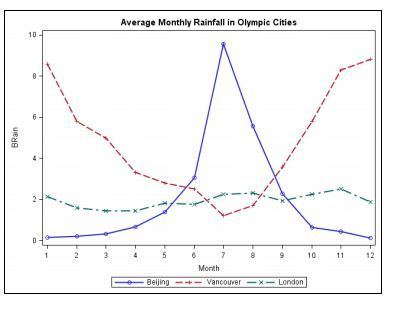## proc sgplot symbols option

I am using proc sgplot and i need sybols in graph as for one treatment as 1 and other treatment as 2 and other treatment as P.

How can i do it using sgplot

9 REPLIES 9DanH_sas
SAS Super FREQ

## Re: proc sgplot symbols option

What version of SAS are you using?

## Re: proc sgplot symbols option

SAS 9.4DanH_sas
SAS Super FREQ

## Re: proc sgplot symbols option

Are you scatter plots grouped or overlaid? It might be helpful to see a snippit of the SGPLOT code.

## Re: proc sgplot symbols option

Attached a layout. in this instaed of circle i need '1' and instead of '*' i need as '2' and other one as 'P' may be.DanH_sas
SAS Super FREQ

## Re: proc sgplot symbols option

The reason I need to know if your SGPLOT code is using groups versus overlays is that it will make a difference in the solution. Is your plot statement using the GROUP option, or are you using three plot statements?

## Re: proc sgplot symbols option

In 9.4 there is an option symbol=, for which you can put a character in to display for each plot.  See this:

http://blogs.sas.com/content/graphicallyspeaking/?s=symbol%3D

Note that blog is very useful for any graph questions.DanH_sas
SAS Super FREQ

## Re: proc sgplot symbols option

Here is a sample solution both the GROUPED and OVERLAID cases:

Overlaid:

proc sgplot data=sashelp.class;

symbolchar name=plot1 char='0031'x;

symbolchar name=plot2 char='0032'x;

scatter x=age y=height / markerattrs=(symbol=plot1 size=12pt);

scatter x=age y=height / markerattrs=(symbol=plot2 size=12pt);

run;

Grouped (two solutions):

ods graphics / attrpriority=none;

proc sgplot data=sashelp.class;

symbolchar name=plot1 char='0031'x;

symbolchar name=plot2 char='0032'x;

styleattrs datasymbols=(plot1 plot2);

scatter x=age y=height / group=sex markerattrs=(size=12pt);

run;

- OR -

data attrmap;

retain id "gender";

input value \$ markersymbol \$;

cards;

F plot1

M plot2

;

run;

proc sgplot data=sashelp.class dattrmap=attrmap;

symbolchar name=plot1 char='0031'x;

symbolchar name=plot2 char='0032'x;

scatter x=age y=height / group=sex markerattrs=(size=12pt) attrid=gender;

run;

## Re: proc sgplot symbols option

1. Produce a simple scatter plot 2. Label the axes 3. Label the data points 4. Group the data points using colors and symbols SESUG 2011 2 5. Use the %MODSTYLE macro to set non-default colors and symbols 6. Maintain the same colors and symbols across plots 7. Add a 45-degree line 8. Change the color of the 45-degree line 9. Label the legend for the colors and symbols 10. Position the legend 11. Adjust the height and width of the plot 12. Specify the output location.

## Re: proc sgplot symbols option

1. Produce a simple scatter plot 2. Label the axes 3. Label the data points 4. Group the data points using colors and symbols SESUG 2011 2 5. Use the %MODSTYLE macro to set non-default colors and symbols 6. Maintain the same colors and symbols across plots 7. Add a 45-degree line 8. Change the color of the 45-degree line 9. Label the legend for the colors and symbols 10. Position the legend 11. Adjust the height and width of the plot 12. Specify the output location
Discussion stats
• 9 replies
• 3176 views
• 0 likes
• 4 in conversation